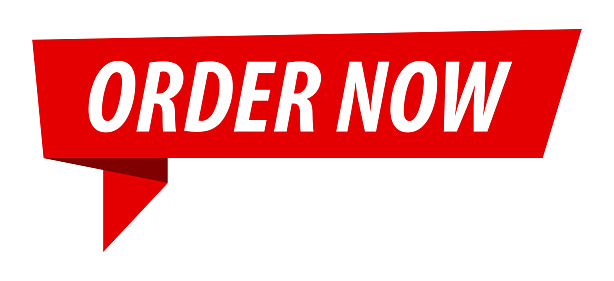# Essay: Demand Analysis; An Example

7 Dec

## Essay: Demand Analysis; An Example

Sample Essay

Clean Supreme is a company that manufactures and sells powdered laundry detergent in the United States. The firm has estimated the following regression equation for the demand of its Brand Z detergent:

QZ = 1.0 – 2.0PZ + 0.7I + 1.5PA + 0.5PB + 1.5A

Where QZ = sales of powdered laundry detergent brand Z, in millions of boxes per year

PZ = price of powdered laundry detergent brand Z, in dollars per box

I = personal disposable income, in trillions of dollars per year
PA = price of the competitive brand of powdered laundry detergent, in dollars per box
PB = price of liquid laundry detergent, in dollars per bottle
A = advertising expenses for powdered laundry detergent brand Z, in thousands of dollars per year

Question 1

For the current year, assume that PZ = \$6, I = 2.3, PA = \$6.25, PB = \$4.50, and A = \$1.5. What is the expected demand of the Brand Z detergent for this year?

When, PZ = \$6

I = 2.3

PA – \$6.25

PB = \$4.50

A = 1.5

Then, using the regression equation for demand:

QZ = 1.0 – 2.0PZ + 0.7I + 1.5PA + 0.5PB + 1.5A

QZ = 1.0 – 2.0 (6) + 0.7 (2.3) + 1.5 (6.25) + 0.5 (4.5) + 1.5 (1.5)

QZ = 4.485

Therefore, the demand for the detergent is 4.485

These are just excerpts of essays for you to view. Please click on Order Now for custom essays, research papers, term papers, thesis, dissertations, case studies and book reports.